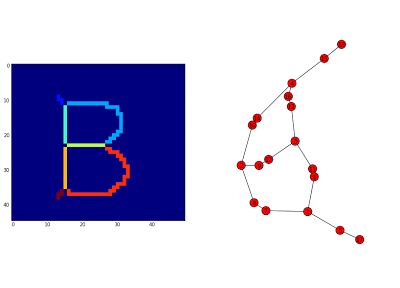Wednesday, June 5, 2013

From binary image skeleton to networkx graph (work in progress ...)

To recognize objects in an image methods involving graphs have been developped as the shock graph method. These graphs are derived from image skeleton and then handled, compared. However, it seems taken for granted that a graph is available for work.
Here is proposed a python code generating a networkx graph object from the skeleton of a binary image.

The image of the B letter was chosen as model. The image is generated with PIL:

Skeleton decomposition:

The shape skeleton is computed by morphological thining, branched points are extracted from the skeleton with a hit-or-miss operator and labelled:

Finding the neighborhood of the branched points:

• The end points are extracted and labelled from the initial skeleton (top left image) .
• The edges are obtained by difference between the skeleton of the shape and the branched points of the skeleton. Seven edges were found and labelled from 1 to 7 (middle left image).
• The edges are pruned by one pixel, the labels values are 1,2,3,4,5,6,7 (middle right image).
• The edges end points are then extracted and labelled individually (1,2,3,...,14) (bottom left image).
• The end points are also labelled according to their edge of origin ( 1,2,3,4,5,6,7), (bottom right image).
For each branched point, its neighborhood is isolated to get the labels of the adjacent end points (right images):

Building the graph:

The graph is build by adding branched points first (vertex 1, 2, 3, 4), it assumes that at least one branched point exists, then  end points adjacent to the branched points are added (vertex 8,9,10 and 11,12,13 and 14,15,16):
The remaining lonely end points are added to the graph (vertex 17, 18):
The end points are linked using the pruned edges, the edges are weighted according to their pixel size:Graph of the letter B, without initial skeleton pruning. The edges length here do not match to their weight

A bug somewhere (update fri 06, 2013):

For some shapes, as 'Y':
The algorithm fails at the end points linking step. A way to cure the situation is to prune the initial skeleton of 'Y' once:

In the case of the letter 'A', the skeleton must be pruned three times for the algorithm to succeed:

Bug origin:

With the letter 'Y', with initial skeleton pruning, the algorithm yields the following graph:Branched points are figured in red circles on the image of labelled edges and end points are figured by a white star.
In the case of the letter 'A', the algorithm yields :
The bug seems to come from too short edges as after three pruning steps removing the small barbs on the top of the letter, the algorithm succeeds in producing a graph